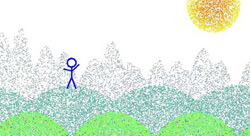Customer Stories ›

# Art Inspires a Lesson in Calculus

"The main advantage of Mathematica is the elegance of its syntax. It lets you write what you think, so the students can concentrate on the mathematical aspects of the exercise rather than on the programming. I think this is the way Mathematica should be used in education. It encourages students to explore, to invent something."

Croatian math and computer science teacher Maja Cvitkovic is not content with the same old math lessons. So when she recently had the opportunity to use Mathematica with a group of teenage students at a workshop in Zagreb, she devised a lesson that gave them a chance to play. The assignment: Create a picture using random dots.

"A common calculus exercise is to present a function and have students plot its graph," explains Cvitkovic. "But this is too passive for me." To help students gain greater insight into functions and their corresponding graphs, Cvitkovic turned the traditional exercise "upside down," as she describes it. Her students began by envisioning an image and then were asked to find the function that would create that image when applied to a list of random dots.

After settling on and sketching an image on paper, students identified where the points required to create that image would be more dense and where they would be more sparse. The next step is where experimentation began: Using what they already knew about the shapes represented by basic equations and simple transformations, students wrote functions in Mathematica and modified them until they found the exact expression for the function that would fit the desired curves and distribution of dots. Then they applied the function to a list of random dots to visualize their pointillist creations. The final stages involved applying colors and replacing dots with different-sized disks if they chose to do so.

Cvitkovic feels the exercise is appropriate for beginning calculus students, also exposing them to a bit of probability theory. It requires knowledge of what happens when two functions are combined and what results when a function is multiplied by a number. In terms of Mathematica skills, the prerequisite for this exercise is simply a basic familiarity with the system, including knowing how to perform calculations, work with lists, and plot graphs of elementary functions. Cvitkovic's students had about 10 two-hour sessions with Mathematica before working on this assignment.

Cvitkovic reports that students enjoy this exercise because it is different and involves creativity. It is motivating because the problem with its "answer" is unique for each student, and it can be repeated to discover endless variations, images, and behaviors of functions.

Cvitkovic finds Mathematica uniquely appropriate for this exercise because it gives students easy access to all of the necessary tools. It takes just a few keystrokes to plot a graph of a function, calculate values for points, and combine parts of a picture. And, she adds, "When they need help, there is excellent online help, complete with examples."

"The main advantage of Mathematica is the elegance of its syntax. It lets you write what you think, so the students can concentrate on the mathematical aspects of the exercise rather than on the programming. I think this is the way Mathematica should be used in education. It encourages students to explore, to invent something."

For her teacher colleagues, Cvitkovic's final thoughts on the topic include encouragement and one caveat: "Just try it! But be warned--you and your students can get addicted to playing with Mathematica."